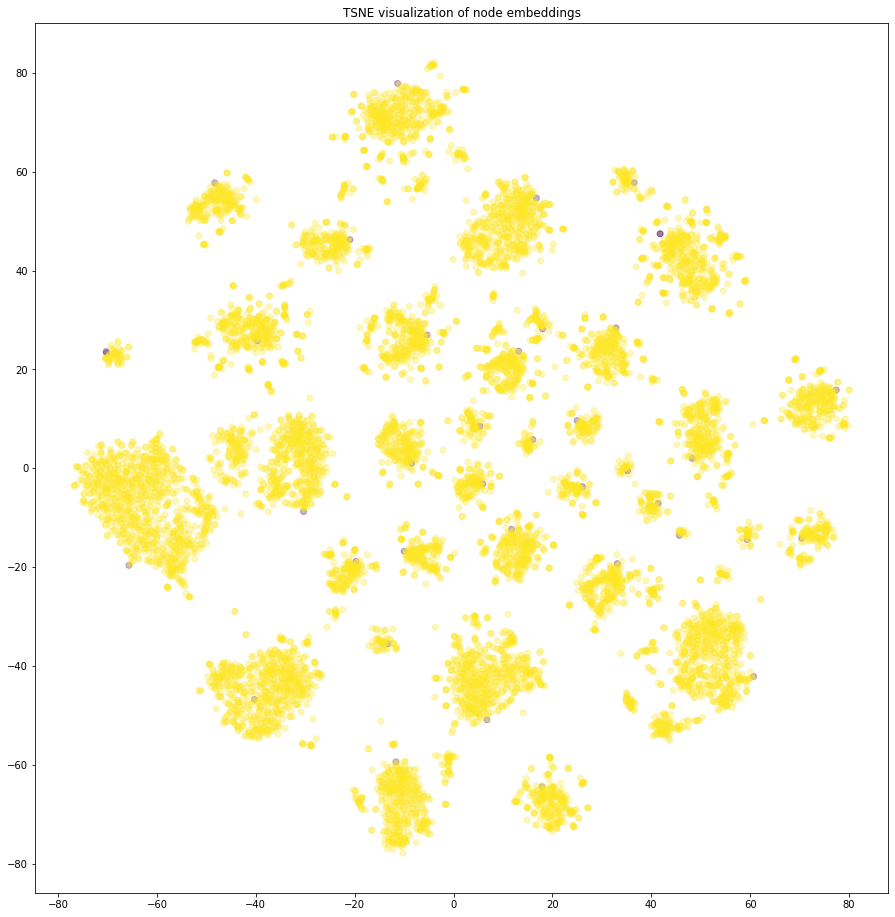# Node representation learning with Metapath2Vec¶

An example of implementing the Metapath2Vec representation learning algorithm using components from the stellargraph and gensim libraries.

References

1. Metapath2Vec: Scalable Representation Learning for Heterogeneous Networks. Yuxiao Dong, Nitesh V. Chawla, and Ananthram Swami. ACM SIGKDD International Conference on Knowledge Discovery and Data Mining (KDD), 135–144, 2017. (link)

2. Distributed representations of words and phrases and their compositionality. T. Mikolov, I. Sutskever, K. Chen, G. S. Corrado, and J. Dean. In Advances in Neural Information Processing Systems (NIPS), pp. 3111-3119, 2013. (link)

3. Gensim: Topic modelling for humans. (link)

4. Social Computing Data Repository at ASU [http://socialcomputing.asu.edu]. R. Zafarani and H. Liu. Tempe, AZ: Arizona State University, School of Computing, Informatics and Decision Systems Engineering. 2009.

:

import matplotlib.pyplot as plt
from sklearn.manifold import TSNE
from sklearn.decomposition import PCA
import os
import networkx as nx
import numpy as np
import pandas as pd
from stellargraph import datasets
from IPython.display import display, HTML

%matplotlib inline


:

dataset = datasets.BlogCatalog3()
display(HTML(dataset.description))
print(
"Number of nodes {} and number of edges {} in graph.".format(
g.number_of_nodes(), g.number_of_edges()
)
)

This dataset is crawled from a social blog directory website BlogCatalog http://www.blogcatalog.com and contains the friendship network crawled and group memberships.
Number of nodes 10351 and number of edges 348459 in graph.


## The Metapath2Vec algorithm¶

The Metapath2Vec algorithm introduced in  is a 2-step representation learning algorithm. The two steps are:

1. Use uniform random walks to generate sentences from a graph. A sentence is a list of node IDs. The set of all sentences makes a corpus. The random walk is driven by a metapath that defines the node type order by which the random walker explores the graph.

2. The corpus is then used to learn an embedding vector for each node in the graph. Each node ID is considered a unique word/token in a dictionary that has size equal to the number of nodes in the graph. The Word2Vec algorithm  is used for calculating the embedding vectors.

## Corpus generation using random walks¶

The stellargraph library provides an implementation for uniform, first order, random walks as required by Metapath2Vec. The random walks have fixed maximum length and are controlled by the list of metapath schemas specified in parameter metapaths.

A metapath schema defines the type of node that the random walker is allowed to transition to from its current location. In the stellargraph implementation of metapath-driven random walks, the metapath schemas are given as a list of node types under the assumption that the input graph is not a multi-graph, i.e., two nodes are only connected by one edge type.

See  for a detailed description of metapath schemas and metapath-driven random walks.

For the BlogCatalog3 dataset we use the following 3 metapaths.

• “user”, “group”, “user”

• “user”, “group”, “user”, “user”

• “user”, “user”

:

walk_length = 100  # maximum length of a random walk to use throughout this notebook

# specify the metapath schemas as a list of lists of node types.
metapaths = [
["user", "group", "user"],
["user", "group", "user", "user"],
["user", "user"],
]

:

from stellargraph.data import UniformRandomMetaPathWalk

# Create the random walker
rw = UniformRandomMetaPathWalk(g)

walks = rw.run(
nodes=list(g.nodes()),  # root nodes
length=walk_length,  # maximum length of a random walk
n=1,  # number of random walks per root node
metapaths=metapaths,  # the metapaths
)

print("Number of random walks: {}".format(len(walks)))

Number of random walks: 30936


## Representation Learning using Word2Vec¶

We use the Word2Vec  implementation in the free Python library gensim  to learn representations for each node in the graph.

We set the dimensionality of the learned embedding vectors to 128 as in .

:

from gensim.models import Word2Vec

model = Word2Vec(walks, size=128, window=5, min_count=0, sg=1, workers=2, iter=1)

:

model.wv.vectors.shape  # 128-dimensional vector for each node in the graph

:

(10351, 128)


## Visualise Node Embeddings¶

We retrieve the Word2Vec node embeddings that are 128-dimensional vectors and then we project them down to 2 dimensions using the t-SNE algorithm.

:

# Retrieve node embeddings and corresponding subjects
node_ids = model.wv.index2word  # list of node IDs
node_embeddings = (
model.wv.vectors
)  # numpy.ndarray of size number of nodes times embeddings dimensionality
node_targets = [g.node_type(node_id) for node_id in node_ids]


Transform the embeddings to 2d space for visualisation

:

transform = TSNE  # PCA

trans = transform(n_components=2)
node_embeddings_2d = trans.fit_transform(node_embeddings)

:

# draw the points
label_map = {l: i for i, l in enumerate(np.unique(node_targets))}
node_colours = [label_map[target] for target in node_targets]

plt.figure(figsize=(20, 16))
plt.axes().set(aspect="equal")
plt.scatter(node_embeddings_2d[:, 0], node_embeddings_2d[:, 1], c=node_colours, alpha=0.3)
plt.title("{} visualization of node embeddings".format(transform.__name__))
plt.show()